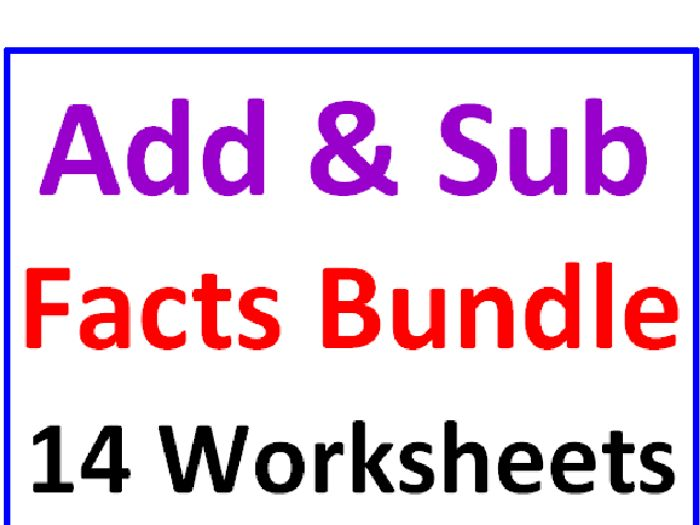ADDITION AND SUBTRACTION FACTS BUNDLE 14 PAGES OF PRACTICE

How important are addition and subtraction facts to your students? This is a complete set of 14 worksheets all dealing with their facts. Here is what you’ll receive:

Pages 1 and 2 – Missing Math Facts (addition only); students are given small equations with a fact missing; they must fill in blanks with the correct facts to make true statements; (30 examples with 6 BONUS questions at the end);

Pages 3 and 4 – Circle The Largest Fact! (addition and subtraction combined); students are given sets of 3 facts; they must first SOLVE each fact and then CIRCLE the largest answer in each set; (12 sets of 3 = 36 facts to solve in all);

Pages 5 – 7 : Greater Than Less Than! (addition and subtraction combined); students use the symbols < and > as they solve their facts in equations; page 5 is all addition, page 6 is all subtraction and page 7 is a combination of both; (42 examples in all); Thinking Skills required! Great practice with Greater Than and Less Than;

Pages 8 – 11 : Match The Facts! (addition and subtraction); students are given ONE fact to solve on the left and FOUR other facts to solve on the right; they must circle the fact from the right that matches the first one on the left, IF there is one; pages 8 and 9 are all addition, pages 10 and 11 all subtraction; (24 examples with 5 facts to solve in EACH example = 120 facts!); time consuming yet effective;

Pages 12 – 14 : Word Problems! (BOTH operations); students must read word problems on the first two pages and write the fact that goes along with them; on the last page students must place their own numbers in boxes and WRITE a word problem to fit their numbers, one for addition one for subtraction;

A COMPLETE set of worksheets for your unit on addition and subtraction facts!

Designed and created by David Filipek.
Permission for downloads to copy for single classroom use only.
Electronic distribution limited to single classroom use only. Not for public display.
\$5.00
Save for later

### Info

Created: Jan 7, 2017

Updated: Feb 22, 2018

png, 22 KB

png, 23 KB